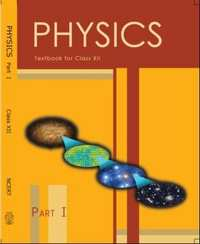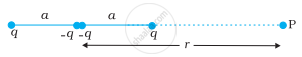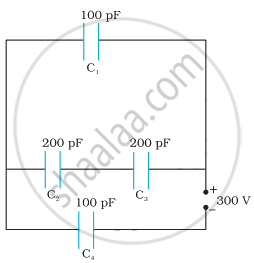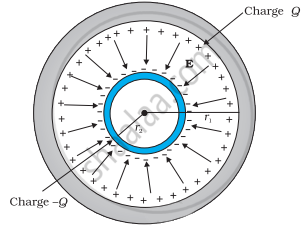# NCERT solutions for Class 12 Physics Textbook chapter 2 - Electrostatic Potential and Capacitance [Latest edition]

#### Chapters## Chapter 2: Electrostatic Potential and Capacitance

Exercise
Exercise [Pages 87 - 92]

### NCERT solutions for Class 12 Physics Textbook Chapter 2 Electrostatic Potential and Capacitance Exercise [Pages 87 - 92]

Exercise | Q 1 | Page 87

Two charges 5 × 10−8 C and −3 × 10−8 C are located 16 cm apart. At what point(s) on the line joining the two charges is the electric potential zero? Take the potential at infinity to be zero.

Exercise | Q 2 | Page 87

A regular hexagon of side 10 cm has a charge 5 µC at each of its vertices. Calculate the potential at the centre of the hexagon.

Exercise | Q 3 | Page 87

Two charges 2 μC and −2 µC are placed at points A and B 6 cm apart.

(a) Identify an equipotential surface of the system.

(b) What is the direction of the electric field at every point on this surface?

Exercise | Q 4 | Page 87

A spherical conductor of radius 12 cm has a charge of 1.6 × 10−7C distributed uniformly on its surface. What is the electric field

(a) Inside the sphere

(b) Just outside the sphere

(c) At a point 18 cm from the centre of the sphere?

Exercise | Q 5 | Page 87

A parallel plate capacitor with air between the plates has a capacitance of 8 pF (1pF = 10−12 F). What will be the capacitance if the distance between the plates is reduced by half, and the space between them is filled with a substance of dielectric constant 6?

Exercise | Q 6 | Page 87

Three capacitors each of capacitance 9 pF are connected in series.

(a) What is the total capacitance of the combination?

(b) What is the potential difference across each capacitor if the combination is connected to a 120 V supply?

Exercise | Q 7 | Page 87

Three capacitors of capacitances 2 pF, 3 pF and 4 pF are connected in parallel.

(a) What is the total capacitance of the combination?

(b) Determine the charge on each capacitor if the combination is connected to a 100 V supply.

Exercise | Q 8 | Page 87

In a parallel plate capacitor with air between the plates, each plate has an area of 6 × 10−3 m2 and the distance between the plates is 3 mm. Calculate the capacitance of the capacitor. If this capacitor is connected to a 100 V supply, what is the charge on each plate of the capacitor?

Exercise | Q 10 | Page 88

A 12 pF capacitor is connected to a 50 V battery. How much electrostatic energy is stored in the capacitor?

Exercise | Q 11 | Page 88

A 600 pF capacitor is charged by a 200 V supply. It is then disconnected from the supply and is connected to another uncharged 600 pF capacitor. How much electrostatic energy is lost in the process?

Exercise | Q 12 | Page 88

A charge of 8 mC is located at the origin. Calculate the work done in taking a small charge of −2 × 10−9 C from a point P (0, 0, 3 cm) to a point Q (0, 4 cm, 0), via a point R (0, 6 cm, 9 cm).

Exercise | Q 14 | Page 88

Two tiny spheres carrying charges 1.5 μC and 2.5 μC are located 30 cm apart. Find the potential and electric field:

(a) at the mid-point of the line joining the two charges, and

(b) at a point 10 cm from this midpoint in a plane normal to the line and passing through the mid-point.

Exercise | Q 15 | Page 88

A spherical conducting shell of inner radius r1 and outer radius r2 has a charge Q.

(a) A charge q is placed at the centre of the shell. What is the surface charge density on the inner and outer surfaces of the shell?

(b) Is the electric field inside a cavity (with no charge) zero, even if the shell is not spherical, but has any irregular shape? Explain.

Exercise | Q 16 | Page 88

(a) Show that the normal component of electrostatic field has a discontinuity from one side of a charged surface to another given by

(vec"E"_2 - vec"E"_1).hat"n" = sigma/in_0

Where hat"n" is a unit vector normal to the surface at a point and σ is the surface charge density at that point. (The direction of hat"n" is from side 1 to side 2.) Hence show that just outside a conductor, the electric field is σ hat"n"/in_0

(b) Show that the tangential component of electrostatic field is continuous from one side of a charged surface to another.

[Hint: For (a), use Gauss’s law. For, (b) use the fact that work done by electrostatic field on a closed loop is zero.]

Exercise | Q 17 | Page 88

A long charged cylinder of linear charged density λ is surrounded by a hollow co-axial conducting cylinder. What is the electric field in the space between the two cylinders?

Exercise | Q 18 | Page 88

In a hydrogen atom, the electron and proton are bound at a distance of about 0.53 Å:

(a) Estimate the potential energy of the system in eV, taking the zero of the potential energy at infinite separation of the electron from proton.

(b) What is the minimum work required to free the electron, given that its kinetic energy in the orbit is half the magnitude of potential energy obtained in (a)?

(c) What are the answers to (a) and (b) above if the zero of potential energy is taken at 1.06 Å separation?

Exercise | Q 19 | Page 90

If one of the two electrons of a Hmolecule is removed, we get a hydrogen molecular ion "H"_2^+. In the ground state of an "H"_2^+, the two protons are separated by roughly 1.5 Å, and the electron is roughly 1 Å from each proton. Determine the potential energy of the system. Specify your choice of zero potential energy.

Exercise | Q 20 | Page 90

Two charged conducting spheres of radii a and b are connected to each other by a wire. What is the ratio of electric fields at the surfaces of the two spheres? Use the result obtained to explain why charge density on the sharp and pointed ends of a conductor is higher than on its flatter portions.

Exercise | Q 21 | Page 90

Two charges −q and +q are located at points (0, 0, −a) and (0, 0, a), respectively.

(a) What is the electrostatic potential at the points?

(b) Obtain the dependence of potential on the distance r of a point from the origin when r/a >> 1.

(c) How much work is done in moving a small test charge from the point (5, 0, 0) to (−7, 0, 0) along the x-axis? Does the answer change if the path of the test charge between the same points is not along the x-axis?

Exercise | Q 22 | Page 90

Figure shows a charge array known as an electric quadrupole. For a point on the axis of the quadrupole, obtain the dependence of potential on r for r/a >> 1, and contrast your results with that due to an electric dipole, and an electric monopole (i.e., a single charge).Exercise | Q 23 | Page 90

An electrical technician requires a capacitance of 2 µF in a circuit across a potential difference of 1 kV. A large number of 1 µF capacitors are available to him each of which can withstand a potential difference of not more than 400 V. Suggest a possible arrangement that requires the minimum number of capacitors.

Exercise | Q 24 | Page 90

What is the area of the plates of a 2 F parallel plate capacitor, given that the separation between the plates is 0.5 cm? [You will realize from your answer why ordinary capacitors are in the range of µF or less. However, electrolytic capacitors do have a much larger capacitance (0.1 F) because of very minute separation between the conductors.]

Exercise | Q 25 | Page 90

Obtain the equivalent capacitance of the network in Figure. For a 300 V supply, determine the charge and voltage across each capacitor.Exercise | Q 26 | Page 90

The plates of a parallel plate capacitor have an area of 90 cm2 each and are separated by 2.5 mm. The capacitor is charged by connecting it to a 400 V supply.

(a) How much electrostatic energy is stored by the capacitor?

(b) View this energy as stored in the electrostatic field between the plates, and obtain the energy per unit volume u. Hence arrive at a relation between u and the magnitude of electric field E between the plates.

Exercise | Q 27 | Page 90

A 4 µF capacitor is charged by a 200 V supply. It is then disconnected from the supply and is connected to another uncharged 2 µF capacitors. How much electrostatic energy of the first capacitor is lost in the form of heat and electromagnetic radiation?

Exercise | Q 28 | Page 90

Show that the force on each plate of a parallel plate capacitor has a magnitude equal to (1/2) QE, where Q is the charge on the capacitor, and E is the magnitude of the electric field between the plates. Explain the origin of the factor 1/2.

Exercise | Q 29 | Page 90

A spherical capacitor consists of two concentric spherical conductors, held in position by suitable insulating supports. Show that the capacitance of a spherical capacitor is given by

C = (4piin_0"r"_1"r"_2)/("r"_1 - "r"_2)where r1 and r2 are the radii of outer and inner spheres, respectively.

Exercise | Q 30 | Page 91

A spherical capacitor has an inner sphere of radius 12 cm and an outer sphere of radius 13 cm. The outer sphere is earthed and the inner sphere is given a charge of 2.5 µC. The space between the concentric spheres is filled with a liquid of dielectric constant 32.

(a) Determine the capacitance of the capacitor.

(b) What is the potential of the inner sphere?

(c) Compare the capacitance of this capacitor with that of an isolated sphere of radius 12 cm. Explain why the latter is much smaller.

Exercise | Q 31.1 | Page 91

Two large conducting spheres carrying charges Q1 and Q2 are brought close to each other. Is the magnitude of the electrostatic force between them exactly given by Q1 Q2/4π∈02, where r is the distance between their centres?

Exercise | Q 31.2 | Page 91

If Coulomb’s law involved 1/r3 dependence (instead of 1/r2), would Gauss’s law be still true?

Exercise | Q 31.3 | Page 91

A small test charge is released at rest at a point in an electrostatic field configuration. Will it travel along the field line passing through that point?

Exercise | Q 31.4 | Page 91

What is the work done by the field of a nucleus in a complete circular orbit of the electron? What if the orbit is elliptical?

Exercise | Q 31.5 | Page 91

We know that electric field is discontinuous across the surface of a charged conductor. Is electric potential also discontinuous there?

Exercise | Q 31.6 | Page 91

What meaning would you give to the capacitance of a single conductor?

Exercise | Q 31.7 | Page 91

Guess a possible reason why water has a much greater dielectric constant (= 80) than say, mica (= 6).

Exercise | Q 32 | Page 91

A cylindrical capacitor has two co-axial cylinders of length 15 cm and radii 1.5 cm and 1.4 cm. The outer cylinder is earthed and the inner cylinder is given a charge of 3.5 µC. Determine the capacitance of the system and the potential of the inner cylinder. Neglect end effects (i.e., bending of field lines at the ends).

Exercise | Q 33 | Page 91

A parallel plate capacitor is to be designed with a voltage rating 1 kV, using a material of dielectric constant 3 and dielectric strength about 107 Vm−1. (Dielectric strength is the maximum electric field a material can tolerate without breakdown, i.e., without starting to conduct electricity through partial ionisation.) For safety, we should like the field never to exceed, say 10% of the dielectric strength. What minimum area of the plates is required to have a capacitance of 50 pF?

Exercise | Q 34 | Page 91

Describe schematically the equipotential surfaces corresponding to

(a) a constant electric field in the z-direction,

(b) a field that uniformly increases in magnitude but remains in a constant (say, z) direction,

(c) a single positive charge at the origin, and

(d) a uniform grid consisting of long equally spaced parallel charged wires in a plane.

Exercise | Q 35 | Page 92

In a Van de Graaff type generator a spherical metal shell is to be a 15 × 106 V electrode. The dielectric strength of the gas surrounding the electrode is 5 × 107 Vm−1. What is the minimum radius of the spherical shell required? (You will learn from this exercise why one cannot build an electrostatic generator using a very small shell which requires a small charge to acquire a high potential.)

Exercise | Q 36 | Page 92

A small sphere of radius r1 and charge q1 is enclosed by a spherical shell of radius r2 and charge q2. Show that if q1 is positive, charge will necessarily flow from the sphere to the shell (when the two are connected by a wire) no matter what the charge q2 on the shell is.

Exercise | Q 37.1 | Page 92

The top of the atmosphere is at about 400 kV with respect to the surface of the earth, corresponding to an electric field that decreases with altitude. Near the surface of the earth, the field is about 100 Vm−1. Why then do we not get an electric shock as we step out of our house into the open? (Assume the house to be a steel cage so there is no field inside!)

Exercise | Q 37.2 | Page 92

A man fixes outside his house one evening a two metre high insulating slab carrying on its top a large aluminium sheet of area 1 m2. Will he get an electric shock if he touches the metal sheet next morning?

Exercise | Q 37.3 | Page 92

The discharging current in the atmosphere due to the small conductivity of air is known to be 1800 A on an average over the globe. Why then does the atmosphere not discharge itself completely in due course and become electrically neutral? In other words, what keeps the atmosphere charged?

Exercise | Q 37.4 | Page 92

What are the forms of energy into which the electrical energy of the atmosphere is dissipated during a lightning?
(Hint: The earth has an electric field of about 100 Vm−1 at its surface in the downward direction, corresponding to a surface charge density = −10−9 C m−2. Due to the slight conductivity of the atmosphere up to about 50 km (beyond which it is good conductor), about + 1800 C is pumped every second into the earth as a whole. The earth, however, does not get discharged since thunderstorms and lightning occurring continually all over the globe pump an equal amount of negative charge on the earth.)

## Chapter 2: Electrostatic Potential and Capacitance

Exercise## NCERT solutions for Class 12 Physics Textbook chapter 2 - Electrostatic Potential and Capacitance

NCERT solutions for Class 12 Physics Textbook chapter 2 (Electrostatic Potential and Capacitance) include all questions with solution and detail explanation. This will clear students doubts about any question and improve application skills while preparing for board exams. The detailed, step-by-step solutions will help you understand the concepts better and clear your confusions, if any. Shaalaa.com has the CBSE Class 12 Physics Textbook solutions in a manner that help students grasp basic concepts better and faster.

Further, we at Shaalaa.com provide such solutions so that students can prepare for written exams. NCERT textbook solutions can be a core help for self-study and acts as a perfect self-help guidance for students.

Concepts covered in Class 12 Physics Textbook chapter 2 Electrostatic Potential and Capacitance are Combination of Capacitors, Capacitors and Capacitance, Dielectrics and Polarisation, Free Charges and Bound Charges Inside a Conductor, Conductors and Insulators Related to Electric Field, Electrical Potential Energy of a System of Two Point Charges and of Electric Dipole in an Electrostatic Field, Equipotential Surfaces, Potential Due to a System of Charges, Electric Potential Difference, Potential Due to a Point Charge, Electric Potential, Van De Graaff Generator, Effect of Dielectric on Capacity, The Parallel Plate Capacitor, Electrostatics of Conductors, Potential Energy of a Dipole in an External Field, Potential Energy of a System of Two Charges in an External Field, Potential Energy of a Single Charge, Potential Energy of a System of Charges, Potential Due to an Electric Dipole, Relation Between Electric Field and Electrostatic Potential, Energy Stored in a Capacitor, Capacitance of a Parallel Plate Capacitor with and Without Dielectric Medium Between the Plates.

Using NCERT Class 12 solutions Electrostatic Potential and Capacitance exercise by students are an easy way to prepare for the exams, as they involve solutions arranged chapter-wise also page wise. The questions involved in NCERT Solutions are important questions that can be asked in the final exam. Maximum students of CBSE Class 12 prefer NCERT Textbook Solutions to score more in exam.

Get the free view of chapter 2 Electrostatic Potential and Capacitance Class 12 extra questions for Class 12 Physics Textbook and can use Shaalaa.com to keep it handy for your exam preparation